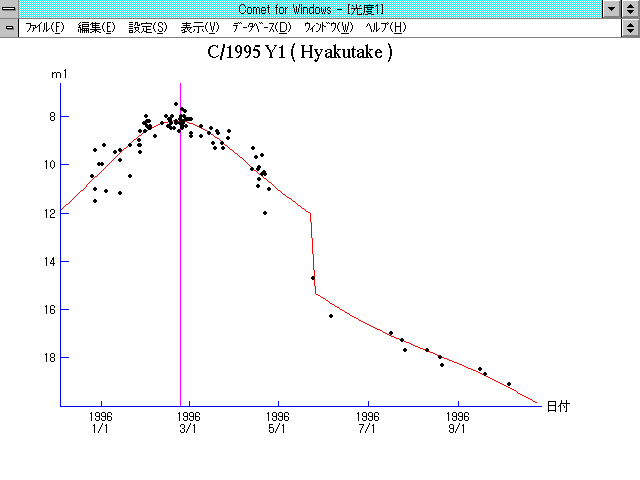# \$BI4IpWB@1(B

C/1995 Y1 ( Hyakutake )###\$B%W%m%U%#!<%k(B

 \$BH/8+F|(B 1995\$BG/(B12\$B7n(B25\$BF|(B \$BH/8+8wEY(B 10.5\$BEy(B \$BH/8+ \$BI4IpM5;J(B (\$BF|K\(B)

###\$B50F;MWAG(B

```   The following improved orbital elements, by Kenji Muraoka, are from
240 observations 1995 Dec. 26 to 1996 Sept. 21, including 9 Planets,
Moon and 5 minor planets perturbations and non-gravitational effect
of style II.    The mean residual is +/- 0.72 arc seconds.

Epoch  =  1996 Feb.  7.0  TT       JDT = 2450120.5
T  =  1996 Feb. 24.28741       +/- 0.00030 (m.e.) TT
Peri. =   46.34885                +/- 0.00050
Node  =  195.76126                +/- 0.00011   (2000.0)
Incl. =   54.46586                +/- 0.00005
q  =    1.0546139              +/- 0.0000058 AU
e  =    1.0002436              +/- 0.0000131
1/a  =   -0.0002310              +/- 0.0000125 1/AU
A1  =   +0.49                   +/- 0.20
A2  =   -0.9498                 +/- 0.0701
```

###\$B@1?^(B1995\$BG/(B 8\$B7n(B 1\$BF|!A(B1996\$BG/(B 2\$B7n(B27\$BF|(B1995\$BG/(B11\$B7n(B20\$BF|!A(B1996\$BG/(B10\$B7n(B 4\$BF|(B

###\$B8wEYJQ2=(B

```        m1 =  7.4 + 5 log\$B&\$(B + 12.5 log r  [  ,90]  (              \$B!A(B1996\$BG/(B 5\$B7n(B24\$BF|(B)
m1 = 10.3 + 5 log\$B&\$(B + 13.5 log r  [90,  ]  (1996\$BG/(B 5\$B7n(B24\$BF|!A(B              )
```##### \$B50F;MWAG\$OB<2,7r<#;a\$N7W;;\$K\$h\$k\$b\$N\$G\$9!#(B \$B@1?^\$O(B StellaNavigator Ver.2.0 for Windows (\$B%"%9%H%m%"!<%D(B \$BJTCx(B / \$B%"%9%-!<=PHG6I4)(B) \$B\$G:n@.\$7\$?\$b\$N\$G\$9!#(B \$B8wEY%0%i%U\$O(BComet for Windows\$B\$G:n@.\$7\$?\$b\$N\$G\$9!#(B